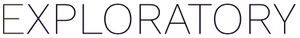# How to access the column name inside of mutate multiple columns step

#1

Suppose you have the following two columns (cola and colb) and for each column, you want to replace “TRUE” with the column name.

To do so, you can use select these columns and select “Replace Values” -> “Set Conditions”

And enter an R script like below. In the script, dot (.) represents a column (as an object) and `deparse(substitute(.))` represents the column name (as string)

``````case_when(
. == "TRUE" ~ deparse(substitute(.)),
TRUE ~ .)
``````

After you run the command, you’ll get the following results.

1 Like